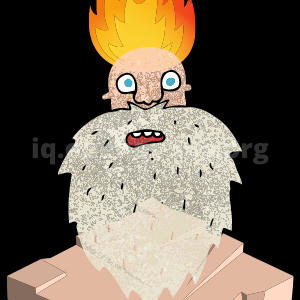×

Search anything:

# Array Interview Questions [MCQ with answers]

#### Data Structures List of Interview Questions Interview Problems on ArrayGet this book -> Problems on Array: For Interviews and Competitive Programming

This is the list of Interview Questions based on Array Data Structure. You must practice these Multiple Choice Questions. This will help you master Array Coding Questions for Interviews at companies like Google and Microsoft.

N-1
N
N/2
logN

## Question 2

#### What is the minimum number of comparisons to find the 2nd largest element provided you have found the largest element?

logN - 1
N-1
N
N + logN - 2
The minimum number of comparisons required to find the 2nd largest element is N + logN - 2. Of this, N-1 comparisons are required to find the largest element. So, only logN - 1 extra comparisons are needed to find the 2nd largest element. To learn more about this, go through this article.

## Question 3

#### In Array Data Structure, what is the biggest limitation?

Pre-defined array size
Slow access time
Slow deletion operation
No random access
The biggest limitation of array is that we need to define the size of array beforehand. This is possible only if the maximum size of array is known beforehand and may lead to memory wastage. The solution is to use Dynamic Array or Linked List.

## Question 4

#### In Dynamic Array, what is the worst case Time Complexity of inserting an new element?

O(N)
O(1)
O(logN)
O(N^2)
The worst case Time Complexity of inserting an new element in a Dynamic Array is O(N). On average, it is O(1). This is because if the array is full and we want to insert a new element, a new array with size 2N is allocated and all N elements are copied before inserting the new element. This makes the worst case O(N).

## Question 5

#### If we have N elements, what is the maximum size of the Dynamic Array?

(N left shift 1) AND (NOT N)
2N
N
N left shift 1
If we have N elements, the maximum size of Dynamic Array is the power of 2 (2^M) which is just greater or equal to N. This is because size of Dynamic Array is always a power of 2 and it increases by doubling.

## Question 6

#### What is the average case Time Complexity to delete a specific element in an array?

O(N)
O(1)
O(logN)
O(loglogN)
The average case Time Complexity to delete a specific element in an array is O(N). This is because on deleting an element, all elements after it are shifted to the left by one position. This allows all elements in the array to stay together and makes the deletion operation O(N).

## Question 7

#### How is an N-dimensional array stored in memory?

1D array
2D array
N-D array
A N-dimensional array is stored as a 1-D array in memory with ordering of row-major or column-major.

## Question 8

#### To move all negative numbers to the front of array, how many traversals are needed?

1
2
N
logN
We can move all negative numbers to the front of the array in just one traversal. To learn the algorithm required to solve this problem, check This article.

## Question 9

#### Array was improved by Dynamic Array. Dynamic Array is improved by?

Hashed Array Tree
Suffix Array
Prefix Sum Array
Bit Array

O(N logN logN)
O(N^2 logN)
O(N^2)
O(N)

## Question 11

#### Using Rolling Hash technique in array, the time complexity to find the hash of a sub-array is?

O(1)
O(N)
O(logN)
O(N logN)
Using Rolling Hash technique in array, the time complexity to find the hash of a sub-array is O(1) with a pre-processing of O(N). To learn more about Rolling Hash, go through this article.

## Question 12

#### Which data structure is used to find the least frequent element in an array?

Hash Map
Sorting
Brute force
Using Hash Map, you can find the least frequent element in an array takes O(N) time and O(N) space. Other approaches are not optimal in terms of time complexity. To learn more, go this algorithm.

## Question 13

#### Which Algorithm is used to find the largest sub-array sum in optimal time O(N)?

Slow Fast pointer
Boyer Moore algorithm
option4

## Question 14

#### Boyer Moore voting algorithm is used to find the majority element among the given sequence of elements in an array which occurs more than N/2 times. What is the Time Complexity provided space complexity is O(1)?

O(N)
O(N logN)
O(N^2)
O(N loglogN)
Boyer Moore voting algorithm is used to find the majority element among the given sequence of elements in an array which occurs more than N/2 times in linear time O(N) with space complexity O(1). To learn more about Boyer Moore Algorithm, go through this article.

## Question 15

#### If there are 1024 elements and we have found the largest element, how many comparisons are needed to find the 2nd largest element?

9
1023
1022
10
If there are 1024 elements and we have found the largest element, we need only 9 comparisons to find the 2nd largest element. 9 = log(1024) - 1. The minimum number of comparisons required to find the 2nd largest element is N + logN - 2. Of this, N-1 comparisons are required to find the largest element. So, only logN - 1 extra comparisons are needed to find the 2nd largest element. To learn more about this, go through this article.

With these questions (with answers) at OpenGenus, you must have a strong idea of Array and good practice of Coding Interview Porblems. Best of luck for your Coding Interview.